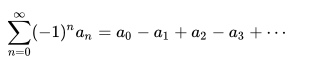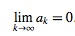# Alternating Series Test / Remainder

Sequence and Series > Alternating Series

## Alternating Series Test

The alternating series test is a simple test we can use to find out whether or not an alternating series converges (settles on a certain number). Basically, if the following things are true, then the series passes the test and shows convergence:

• The terms decrease in absolute value,
• The series eventually decreases; It isn’t necessary that all the terms decrease, just that the overall trend is decreasing.

It goes without saying that you also need to check that the series is actually alternating as well; otherwise, it can’t pass the “alternating” series test.

## What is an Alternating Series?

An alternating series has terms that are alternatively positive and negative. It is a series that can be written in the form:where all the ak are either positive or negative.

It doesn’t matter if the first term is positive or negative, as along as the series alternates.

## Odd and Even Terms

Alternating series can have negative odd or even numbered terms. For example, in the series
-1 + 1/2 – 1/4 + 1/8 – 1/16,
the odd terms (1, 3, 5…) are negative. This can be written as

an = (-1)n bn
bn ≥0

A series might also have negative even terms (i.e. the 2nd, 4th, 6th…), like the geometric series, which has the first few terms
1 – 1/2 + 1/4 – 1/8 + 1/16….
This can be written as:

an = (-1)n+1 bn
bn ≥ 0

## The Alternating Series Test

If a series fails the second requirement for the alternating series test (that the series eventually decreases), that means the series doesn’t converge. However, it doesn’t necessarily mean that the series diverges.

The alternating series simply tells us that the absolute value of each of the terms decreases monotonically, i.e., if a1 ≥ a2 ≥… and ifthe series converges.

## Example of the Alternating Series Test

Suppose we apply the test to the seriesThis series is alternating; the terms alternate between positive and negative. The first three terms of the series increase, but they will add up to a constant, and so don’t effect whether or not the series converges or diverges. To look at what happens as x gets large, we look at the derivativeThis derivative is consistently negative for large x, so we know that the values of ak are on a negative downward slope, i.e., they are constantly decreasing. Therefore, we can conclude by the alternating series test that the series converges.

## Alternating Series Remainder

An alternating series remainder is the difference between our estimation of the series and the actual value.

We never really know what our remainder is, exactly, because we can never tell what our series actually sums to. But our estimations are based on partial sums, and there are several different tests we can use to get estimates of the remainder.

Since it is an estimate of the error in an estimate, it may make more sense to consider the number we are searching for to be a term that bounds the error or remainder, not the error itself.

## How to Estimate an Alternating Series Remainder

If we are looking at the remainder, we’ve decided that our series converges. And if it converges, it satisfies the alternating series test.

This same test gives us a simple way to estimate the remainder. If our series is given byand S represents the sum of the series. We can call the Nth partial sum SN.

Then, for N greater than 1 our remainder will be RN = S – SN and we know that:To find the absolute value of the remainder, then, all you need to do is calculate the N + 1st term in the series. This is usually easy to do if you know your series.

## References

Guichard, David. Alternating Series. From Calculus: Early Transcendentals. Retrieved from https://www.whitman.edu/mathematics/calculus_online/section11.04.html on October 31, 2018